ICSE Class 9 Simultaneous Equations Solution New Pattern

## ICSE Class 9 Simultaneous Equations Solution New Pattern By Clarify Knowledge

ICSE Class 9 Simultaeous Equations Solution New Pattern 2022

## Chapter 1 - Rational and Irrational Numbers Exercise Ex. 6(E)

Question 5

The sum of two numbers is 8 and the difference of their squares is 32. Find the numbers.Solution 5

Let the two numbers be x and y.

Then,

x + y = 8 …..(i)

x2 - y2 = 32 …..(ii)

⟹ (x - y)(x + y) = 32

⟹ x - y = 4 ….. (iii)

Adding (i) and (iii), we get

2x = 12 ⟹ x = 6

From (i), y = 8 - x = 8 - 6 = 2

Therefore, the numbers are 6 and 2.Question 1

The ratio of two numbers is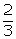. If 2 is subtracted from the first

and 8 from the second, the ratio becomes the reciprocal of the original ratio. Find the numbers.Solution 1

Let the two numbers be x and y

According to the question,

3x - 2y = 0 ...(1)

Also,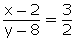2x - 3y = -20 ...(2)

Multiplying equation no. (1) by 2 and (2) by 3and substracting

From (1), we get

3x - 2(12) = 0

x = 8

Thus, the numbers are 8 and 12.Question 2

Two numbers are in the ratio 4 : 7. If thrice the larger be added to twice the smaller, the sum is 59. Find the numbers.Solution 2

Let the smaller number be x

and the larger number bey.

According to the question,

7x -; 4y = 0...(1)

and,3y + 2x = 59...(2)

Multiplying equation no. (1) by 3 and (2) by 4.and adding them

x =From (1)

Hence, the number are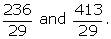Question 3

When the greater of the two numbers increased by 1 divides the sum of the numbers, the result is. When the difference of these numbers is divided by the smaller, the result. Find the numbers.Solution 3

Let the two numbers be a and b respectively such that b > a.

According to given condition,

Question 4

The sum of two positive numbers x and y (x > y) is 50 and the difference of their squares is 720. Find the numbers.Solution 4

Two numbers are x and y such that x > y.

Now,

x + y = 50 ….(i)

And,

y2 - x2 = 720

⇒ (y - x)(y + x) = 720

⇒ (y - x)(50) = 720

⇒ y - x = 14.4 ….(ii)

Adding (i) and (ii), we get

2y = 64.4

⇒ y = 32.2

Substituting the value of y in (i), we have

x + 32.2 = 50

⇒ x = 17.8

Thus, the two numbers are 17.8 and 32.2 respectively.Question 6

Solution 6

Two numbers are x and y respectively such that x > y.

Then,

x - y = 4 ….(i)

⇒ x = 4 + y

And,

Question 7

Two numbers are in the ratio 4:5. If 30 is subtracted from each of the numbers, the ratio becomes 1:2. Find the numbers.Solution 7

Question 8

If the numerator of a fraction is increased by 2 and denominator is decreased by 1, it becomes. If the numerator is increased by 1 and denominator is increased by 2, it becomes. Find the fraction.Solution 8

Let the numerator and denominator a fraction be x and y respectively .

According to the question,

3x - 2y = -8...(1)

And,

Now subtracting,

From (1) ,

3x - 2 (7) = -8

3x = -8 + 14

x = 2

Required fraction =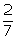Question 9

The sum of the numerator and the denominator of a fraction is equal to 7. Four times the numerator is 8 less than 5 times the denominator. Find the fraction.Solution 9

Let the numerator and denominator of a fraction be x and y respectively .Then the fraction will be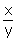According to the question,

x + y = 7...(1)

5y - 4x = 8...(2)

Multiplying equation no. (1) by 4 and add with (2),

From (1)

x + 4 = 7

x = 3

Required fraction =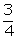Question 10

Solution 10

Question 11

Solution 11

Question 12

The sum of the digits of the digits of two digit number is 5. If the digits are reversed, the number is reduced by 27. Find the number.Solution 12

Let the digit at unit’s place be x and the digit at ten’s place y.

Required no. = 10y + x

If the digit’s are reversed,

Reversed no. = 10y + x

According to the question,

x + y = 5...(1)

and,

(10y + x) - (10x + y) = 27

From (1)

x + 4 = 5

x = 1

Require no is

10 (4) + 1 = 41Question 13

The sum of the digits of a two digit number is 7. If the digits are reversed, the new number decreased by 2, equals twice the original number. Find the number.Solution 13

Let the digit at unit’s place be x and the digit at ten’s place be y.

Required no. = 10y + x

If the digit’s are reversed

Reversed no. = 10x + y

According to the question,

x + y = 7...(1)

and,

10x + y - 2 = 2(10y + x).

8x - 19y = 2...(2)

Multiplying equation no. (1) by 19.

x = 5

From (1)

5 +y = 7

y = 2

Required number is

10(2) + 5

= 25.Question 14

The ten’s digit of a two digit number is three times the unit digit. The sum of the number and the unit digit is 32. Find the number.Solution 14

Let the digit at unit’s place be x and the digit at ten’s place be y.

Required no. = 10y + x

According to the question

y = 3x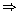3x - y = 0...(1)

and,10y + x + x = 32

10y + 2x = 32...(2)

Multiplying equation no. (1) by 10

From (1),we get

y = 3(1) = 3

Required no is

10(3) + 1 = 31Question 15

A two-digit number is such that the ten’s digit exceeds twice the unit’s digit by 2 and the number obtained by inter-changing the digits is 5 more than the the sum of the digits. Find the two digit number.Solution 15

Let the digit a unit’s place be x and the digit at ten’s place be y.

Required no. = 10y + x.

According to the question,

y - 2x = 2

-2x + y = 2...(1)

and,

(10x + y) -3 (y + x) = 5

7x - 2y = 5...(2)

Multiplying equation no. (1) by 2.

From (1) ,we get

-2(3) + y = 2y = 8

Required number is

10(8) + 3 = 83.Question 16

Four times a certain two digit number is seven times the number obtained on interchanging its digits. If the difference between the digits is 4; find the number.Solution 16

Question 17

The sum of two digit number and the number obtained by interchanging the digits of the number is 121. If the digits of the number differ by 3, find the number.Solution 17

Question 18

A two digit number is obtained by multiplying the sum of the digits by 8. Also, it is obtained by multiplying the difference of the digits by 14 and adding 2. Find the number.Solution 18

## Chapter 6 - Simultaneous (Linear) Equations (Including Problems) Exercise Ex. 6(C)

Question 1

Solve, using cross-multiplication :

4x + 3y = 17

3x - 4y + 6 = 0Solution 1

Question 2

Solve, using cross-multiplication :

3x + 4y = 11

2x + 3y = 8Solution 2

Question 3

Solve, using cross-multiplication :

6x + 7y - 11 = 0

5x + 2y = 13Solution 3

Question 4

Solve, using cross-multiplication :

5x + 4y + 14 = 0

3x = -10 - 4ySolution 4

Question 5

Solve, using cross-multiplication :

x - y + 2 = 0

7x + 9y = 130Solution 5

Question 6

Solve, using cross-multiplication :

4x - y = 5

5y - 4x = 7Solution 6

Question 7

Solve, using cross-multiplication :

4x - 3y = 0

2x + 3y = 18Solution 7

Question 8

Solve, using cross-multiplication :

8x + 5y = 9

3x + 2y = 4Solution 8

Question 9

Solve, using cross-multiplication :

4x - 3y - 11 = 0

6x + 7y - 5 = 0Solution 9

Question 10

Solve, using cross-multiplication :

4x + 6y = 15

3x - 4y = 7Solution 10

Question 11

Solve, using cross-multiplication:

0.4x - 1.5y = 6.5

0.3x + 0.2y = 0.9Solution 11

Question 12

Solve, using cross-multiplication:

Solution 12

## Chapter 6 - Simultaneous (Linear) Equations (Including Problems) Exercise Ex. 6(D)

Question 1

Solution 1

Multiplying equation no. (1) by 7 and (2) by 4.

From (1)

Question 2

Solve the pairs of equations:

Solution 2

Question 3

Solution 3

Question 4

Solution 4

Question 5

Solve:Hence, find 'a' if y = ax + 3.Solution 5

Multiplying equation no. (1) by 5 and (2) by 2.

From (1) 3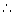y = ax + 3

Question 6

Solve:

Solution 6

(ii)

Question 7

Solve:

(i) x + y = 2xy

x - y = 6xy

(ii) x+ y = 7xy

2x - 3y = -xySolution 7

(i)

From (1)

(ii)

x + y = 7xy...(1)

2x - 3 = -xy...(2)

Multiplying equation no. (1) by 3.

From (1)

Question 8

Solution 8

Question 9

Solution 9

Question 10

Solution 10

## Chapter 6 - Simultaneous (Linear) Equations (Including Problems) Exercise Ex. 6(F)

Question 1

Five years ago, A's age was four times the age of B. Five years hence, A’s age will be twice the age of B. Find their preset ages.Solution 1

Let present age of A = x years

And present age of B = y years

According to the question,

Five years ago,

x - 5 = 4(y - 5)

x - 4y = -15...(1)

Five years later,

x + 5 = 2(y + 5)

Now subtracting (1)from(2)

From (1)

x - 4 (10) = -15

x = 25

Present ages of A and B are 25 years and 10 years respectively.Question 2

A is 20 years older than B. 5 years ago, A was 3 times as old as B. Find their present ages.Solution 2

Let A’s presentage be x years

and B’s present age be y years

According to the question

x = y + 20

x - y = 20...(1)

Five years ago,

x - 5 = 3(y - 5)

Subtracting (1)from(2),

y = 15

From (1)

x = 15 + 20

x = 35

Thus, present ages of A and B are 35 years and 15 years.Question 3

Four years ago, a mother was four times as old as her daughter. Six years later, the mother will be two and a half times as old as her daughter at that time. Find the present ages of mother and her daughter.Solution 3

Question 4

The age of a man is twice the sum of the ages of his two children. After 20 years, his age will be equal to the sum of the ages of his children at that time. Find the present age of the man.Solution 4

Question 5

The annual incomes of A and B are in the ratio 3 : 4 and their annual expenditure are in the ratio 5 : 7. If each Rs. 5000; find their annual incomes.Solution 5

Let A’s annual in come = Rs.x

and B’s annual income = Rs. y

According to the question,

4x - 3y = 0...(1)

and,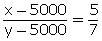7x - 5y = 10000...(2)

Multiplying equation no. (1) by 7 and (2) by 4.and subtracting (4) from (3)

From (1)

4x - 3 (40000) = 0

x = 30000

Thus, A’s income in Rs. 30,000 and B’s income is Rs. 40,000.Question 6

In an examination, the ratio of passes to failures was 4 : 1. Had 30 less appeared and 20 less passed, the ratio of passes to failures would have been 5 : 1. Find the number of students who appeared for the examination.Solution 6

Let the no. of pass candidates be x

and the no. of fail candidates be y.

According to the question,

x - 4y = 0...(1)

and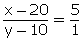x - 5y = -30...(2)

From (1)

- 4(30) = 0

x = 120

Total students appeared = x + y

= 120 + 30

= 150Question 7

A and B both the have some pencils. If A gives 10 pencils to B, then B will have twice as many as A. And if B gives 10 pencils to A, then they will have the same number of pencils. How many pencils does each have?Solution 7

Let the numberof pencils with A = x

and the number of pencils with B = y.

If A gives 10 pencils to B,

y + 10 = 2(x - 10)

2x - y = 30...(1)

If B gives to pencils to A

y - 10 = x + 10

From (1)

2(50) - y = 30

y = 70

Thus, A has 50 pencils and B has 70 pencils.Question 8

1250 persons went to sea a circus-show. Each adult paid Rs. 75 and each child paid Rs. 25 for the admission ticket. Find the number of adults and number of children, if the total collection from them amounts to Rs. 61,250.Solution 8

Let the number of adults = x

and the number of children = y

According to the question,

x + y = 1250...(1)

and 75x + 25y = 61250

x = 6000

From (1)

600 + y = 1250

y = 650

Thus, number of adults = 600

and the number of children = 650.Question 9

Two articles A and B are sold for Rs. 1,167 making 5% profit on A and 7% profiton A and 7% profit on B. IF the two articles are sold for Rs. 1,165, a profit of 7% is made on A and a profit of 5% is made on B. Find the cost prices of each article.Solution 9

Let the cost price of article A = Rs. x

and the cost price of articles B = Rs. y

According to the question,

(x + 5% of x) +(y + 7% of y) = 1167

105x + 107y = 1167...(1)

and107x + 105y = 116500...(2)

212x + 212y = 233200

x + y = 1100...(3)

subtracting (2)from (1)

-2x + 2y = 200

y = 600

from (3)

x +600 = 1100

x = 500

Thus, cost price of article A is Rs. 500.

and that of article B is Rs. 600.Question 10

Pooja and Ritu can do a piece of work indays. If one day work of Pooja be three fourth of one day work of Ritu’ find in how many days each will do the work alone. Solution 10

Let Pooja’s 1 day work =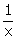and Ritu’s 1 day work =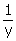According the question,

and,Using the value of y from (2) in (1)

From (2)y = 30

Pooja will complete the work in 40 days and Ritu will complete the work in 30 days.

## Chapter 6 - Simultaneous (Linear) Equations (Including Problems) Exercise Ex. 6(G)

Question 1

Rohit says to Ajay, “Give me hundred, I shall then become twice as rich as you.” Ajay replies, “if you give me ten, I shall be six times as rich as you.” How much does each have originally?Solution 1

Let Rohit has Rs. x

and Ajay has Rs. y

When Ajay gives Rs. 100 to Rohit

x + 100 = 2(y - 100)

x - 2y = -300...(1)

When Rohit gives Rs. 10 to Ajay

6(x-10) = y + 10

6x - y = 70...(2)

Multiplying equation no. (2) By 2.

x = 40

From (1)

40 - 2y = -300-2y = -340y = 170

Thus, Rohit has Rs. 40

and Ajay has Rs. 170Question 2

The sum of a two digit number and the number obtained by reversing the order of the digits is 99. Find the number, if the digits differ by 3.Solution 2

Question 3

Seven times a two digit number is equal to four times the number obtained by reversing the digits. If the difference between the digits is 3 find the number.Solution 3

3Let the digit at ten’s place be x

And the digit at unit’s place be y

Required number = 10x + y

When the digits are interchanged,

Reversed number = 10y + x

According to the question,

7(10x + y) = 4(10y + x)

66x = 33y

2x - y = 0...(1)

Also,

From (1) 2(3) - y = 0

y = 6

Thus, Required number = 10(3) + 6 = 36Question 4

From Delhi station, if we buy 2 tickets for station A and 3 tickets for station B, the total cost is Rs. 77. But if we buy 3 tickets for station A and 5 tickets for station B, the total cost is Rs. 124. What are the fares from Delhi to station A and to station B?Solution 4

Let, the fare of ticket for station A be Rs. x

and the fare of ticket for station B be Rs. y

According, to the question

2x + 3y = 77....(1)

and3x+5y = 124...(2)

Multiplying equation no. (1) by 3 and (2) by 2.

y = 17

From (1) 2x + 3 (17) = 77

2x = 77 - 51

2x = 26

x = 13

Thus, fare for station A = Rs. 13

and, fare for station B = Rs. 17.Question 5

The sum of digit of a two digit number is 11. If the digit at ten's place is increased by 5 and the digit at unit place is decreased by 5, the digits of the number are found to be reversed. Find the original number.Solution 5

Question 6

90% acid solution (90% pure acid and 10% water) and 97% acid solution are mixed to obtain 21 litres of 95% acid solution. How many litres of each solution are mixed.Solution 6

Let the quantity of 90% acid solution be x litres and

The quantity of 97% acid solution be y litres

According to the question,

x + y = 21...(1)

and 90% of x + 97% of y = 95% of 21

90x + 97y = 1995...(2)

Multiplying equation no. (1) by90, we get,

From (1)x + 15 = 21

x = 6

Hence, 90% acid solution is 6 litres and 97% acid solution is 15 litres.Question 7

The class XI students of school wanted to give a farewell party to the outgoing students of class XII. They decided to purchase two kinds of sweets, one costing Rs. 250 per kg and other costing Rs. 350 per kg. They estimated that 40 kg of sweets were needed. If the total budget for the sweets was Rs. 11,800; find how much sweets of each kind were bought?Solution 7

Question 8

Mr. and Mrs. Abuja weight x kg and y kg respectively. They both take a dieting course, at the end of which Mr. Ahuja loses 5 kg and weights as much as his wife weighed before the course.

Mrs. Ahuja loses 4 kg and weighs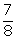th of what her husband weighed before the course. Form two equations in x and y, find their weights before taking the dieting course.Solution 8

Weight of Mr. Ahuja = x kg

and weight of Mrs. Ahuja = y kg.

After the dieting,

x - 5 = y

x - y = 5...(1)

and,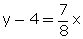7x - 8y = -32...(2)

Multiplying equation no. (1) by 7, we get

Now subtracting (2) from (3)

From (1)

x - 67 = 5x = 72

Thus, weight of Mr. Ahuja = 72 kg.

and that of Mr. Anuja = 67 kg.Question 9

A part of monthly expenses of a family is constants and the remaining vary with the number of members in the family. For a family of 4 person, the total monthly expenses are Rs. 10,400 whereas for a family of 7 persons, the total monthly expenses are Rs. 15,800. Find the constant expenses per month and the monthly expenses of each member of a family.Solution 9

Question 10

The taxi charges in a city consist of a fixed charge together with the charge for the distance covered. For a distance of 10 km, the charge paid is Rs. 315 and for a journey of 15 km, the charge paid is Rs. 465. What are the fixed charges and the charge per kilometer? How much does a person have to pay for travelling a distance of 32 km?Solution 10

Question 11

A lending library has a fixed charge for the first three days and an additional charge for each day thereafter. Geeta paid Rs. 27 for a book kept for seven days, while Mohit paid Rs. 21 for the book he kept for five days. Find the fixed charges and the charge for each extra day.Solution 11

Question 12

The area of a rectangle gets reduced by 9 square units, if its length is reduced by 5 units and breadth is increased by 3 units. However, if the length of this rectangle increases by 3 units and the breadth by 2 units, the area increases by 67 square units. Find the dimensions of the rectangle.Solution 12

Question 13

It takes 12 hours to fill a swimming pool using two pipes. If the pipe of larger diameter is used for 4 hours and the pipe of smaller diameter is used for 9 hours, only half of the pool is filled. How long would each pipe take to fill the swimming pool?Solution 13

## Chapter 6 - Simultaneous Linear Equations Exercise Ex. 6(A)

Question 1

Solve the pairs of linear (simultaneous) equations by the method of elimination by substitution:

8x + 5y = 9

3x + 2y = 4Solution 1

8x + 5y = 9...(1)

3x + 2y = 4...(2)

(2)y =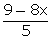Putting this value of y in (2)

3x + 2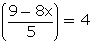15x + 18 - 16x = 20

x = -2

From (1) y =y = 5Question 2

Solve the pairs of linear (simultaneous) equations by the method of elimination by substitution:

2x - 3y = 7

5x + y= 9Solution 2

2x - 3y = 7...(1)

5x + y = 9...(2)

(2)y = 9 - 5x

Putting this value of y in (1)

2x - 3 (9 - 5x) = 7

2x - 27 + 15x = 7

17x = 34

x = 2

From (2)

y = 9 - 5(2)

y = -1Question 3

Solve the pairs of linear (simultaneous) equations by the method of elimination by substitution:

2x + 3y = 8

2x = 2 + 3ySolution 3

2x + 3y = 8...(1)

2x = 2 + 3y...(2)

(2)2x = 2 + 3y

Putting this value of 2x in (1)

2 + 3y + 3y = 8

6y = 6

y = 1

From (2) 2x = 2 + 3 (1)

x =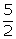x = 2.5Question 4

Solve the following pairs of linear (simultaneous) equations by the method of elimination by substitution:

0.2x + 0.1y = 25

2(x - 2) - 1.6y = 116Solution 4

Question 5

Solve the pairs of linear (simultaneous) equations by the method of elimination by substitution:

6x = 7y + 7

7y - x = 8Solution 5

6x = 7y + 7...(1)

7y - x = 8...(2)

(2)x = 7y - 8

Putting this value of x in (1)

6(7y - 8) = 7y + 7

42y - 48 = 7y + 7

35y = 55

From (2) x =x = 3Question 6

Solve the pairs of linear (simultaneous) equations by the method of elimination by substitution:

y = 4x - 7

16x - 5y = 25Solution 6

y = 4x -7...(1)

16x- 5y = 25...(2)

(1)y = 4x - 7

Putting this value of y in (2)

16x - 5 (4x - 7) = 25

16x - 20x + 35 = 25

-4x = -10

From (1)

y = 10-7=3

Solution is.Question 7

Solve the pairs of linear (simultaneous) equations by the method of elimination by substitution:

2x + 7y = 39

3x + 5y = 31Solution 7

2x + 7y = 39...(1)

3x + 5y = 31...(2)

(1)x =Putting this value of x in (2)

117 - 21y + 10y = 62

-11y = -55

y = 5

From (1) x =Question 8

Solve the following pairs of linear (simultaneous) equations by the method of elimination by substitution:

1.5x + 0.1y = 6.2

3x - 0.4y = 11.2Solution 8

Question 9

Solution 9

Question 10

Solution 10

Question 11

Solve the following pairs of linear (simultaneous) equation using method of elimination by substitution:

3x + 2y =11

2x - 3y + 10 = 0Solution 11

Question 12

Solve the following pairs of linear (simultaneous) equation using method of elimination by substitution:

2x - 3y + 6 = 0

2x + 3y - 18 = 0Solution 12

Question 13

Solve the following pairs of linear (simultaneous) equation using method of elimination by substitution:

Solution 13

Question 14

Solve the following pairs of linear (simultaneous) equation using method of elimination by substitution:

Solution 14

## Chapter 6 - Simultaneous (Linear) Equations (Including Problems) Exercise Ex. 6(B)

Question 1

For solving each pair of equation, in this exercise use the method of elimination by equating coefficients:

13 + 2y = 9x

3y = 7xSolution 1

13 + 2y = 9x...(1)

3y = 7x...(2)

Multiplying equation no. (1) by 3 and (2) by 2, we get,

From (2)

3y = 7(3)

y = 7Question 2

For solving each pair of equation, in this exercise use the method of elimination by equating coefficients:

3x - y = 23

Solution 2

3x - y = 23...(1)

4x + 3y = 48...(2)

Multiplying equation no. (1) by 3

From (1)

3(9) - y = 23

y = 27 - 23

y = 4Question 3

For solving each pair of equation, in this exercise use the method of elimination by equating coefficients:

Solution 3

Question 4

For solving each pair of equation, in this exercise use the method of elimination by equating coefficients:

Solution 4

Multiplying equation no. (1) by 3 and(2) by 5.

From (1)

Question 5

For solving each pair of equation, in this exercise use the method of elimination by equating coefficients:

y = 2x - 6

y = 0Solution 5

y = 2x - 6

y = 0

x = 3 ; y = 0Question 6

For solving each pair of equation, in this exercise use the method of elimination by equating coefficients:

Solution 6

Question 7

For solving each pair of equation, in this exercise use the method of elimination by equating coefficients:

3 - (x - 5) = y + 2

2 (x + y) = 4 - 3ySolution 7

3 - (x - 5) = 4 + 2

2(x + y) = 4 - 3y-x - y = -6x + y = 6...(1)

2x + 5y = 4...(2)

Multiplying equation no. (1) by 2.

From (1)

Question 8

For solving each pair of equation, in this exercise use the method of elimination by equating coefficients:

2x - 3y - 3 = 0

Solution 8

2x -3y - 3 = 02x - 3y = 3...(1)4x + 24y = -3...(2)

Multiplying equation no. (1) by 8.

From (1)

Question 9

For solving each pair of equation, in this exercise use the method of elimination by equating coefficients:

13x+ 11y = 70

11x + 13y = 74Solution 9

13x + 11y = 70...(1)

11x+ 13y = 74...(2)

24x + 24y = 144

x + y = 6...(3)

subtracting (2) from (1)

2x - 2y = -4

x - y = -2...(4)

x + y = 6...(3)

From (3)

2 + y = 6y = 4Question 10

For solving each pair of equation, in this exercise use the method of elimination by equating coefficients:

41x + 53y = 135

53x + 41y = 147Solution 10

41x + 53y = 135...(1)

53x + 41y = 147...(2)

94x + 94y = 282

x + y = 3...(3)

Subtracting (2) from (1)

From (3)

x + 1 = 3x = 2Question 11

If 2x + y = 23 and 4x - y = 19; find the values of x - 3y and 5y - 2x.Solution 11

2x + y = 23...(1)

4x - y = 19...(2)

Adding equation (1) and (2) we get,

2x + y = 23

4x - y = 19

From (1)

2(7) + y = 23

y = 23 - 14y = 9x - 3y = 7 - 3(9) = -20

And 5y - 2x = 5(9) - 2(7) = 45- 14 = 31Question 12

If 10y = 7x - 4 and 12x + 18y = 1; find the values of 4x + 6y and 8y - x.Solution 12

10 y = 7x - 4

-7x + 10y = -4...(1)

12x + 18y = 1...(2)

Multiplying equation no. (1) by 12 and (2) by 7.

From (1)

-7x + 10-7x = -4 +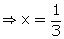4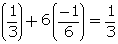and 8y - x = 8Question 13

Solve for x and y:

Solution 13

(i)

(ii)

Question 14

Find the value of m, if x = 2, y = 1 is a solution of the equation 2x + 3y = m.Solution 14

Question 15

10% of x + 20% of y = 24

3x - y = 20Solution 15

Question 16

The value of expression mx - ny is 3 when x = 5 and y = 6. And its value is 8 when x = 6 and y = 5. Find the values of m and n.Solution 16

Question 17

Solve:

11(x - 5) + 10(y - 2) + 54 = 0

7(2x - 1) + 9(3y - 1) = 25Solution 17

Question 18

Solution 18

Question 19

Solution 19

error: Content is protected !!